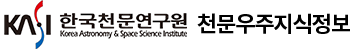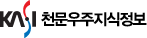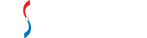#통합검색

#### 통합검색

인기검색어### 한국천문학회지

• 홈으로 이동1968년 ~ 2019년까지 1,094 건한국천문학회지를 격월간 확인하실 수 있습니다.

• The Korean Astronomical Society (The Korean Astronomical Society)
• 계간 (Quarterly)
• ISSN : 1225-4614 (ISSN : 1225-4614)
• DB구축현황 : 1,094건 (DB Construction : 1,094 Articles)
안내사항
총 게시글 1,094 페이지 5/110
41
• Lee, Hee-Jae
• Journal of the Korean astronomical society = 천문학회지
• 50, n.3
• pp.41-49
• 2017
• 원문 바로보기
We conduct BVRI and R band photometric observations of asteroid (5247) Krylov from January 2016 to April 2016 for 51 nights using the Korea Microlensing Telescope Network (KMTNet). The color indices of (5247) Krylov at the light curve maxima are determined as $B-V=0.841{\pm}0.035$ , $V-R=0.418{\pm}0.031$ , and $V-I=0.871{\pm}0.031$ where the phase angle is $14.1^{\circ}$ . They are acquired after the standardization of BVRI instrumental measurements using the ensemble normalization technique. Based on the color indices, (5247) Krylov is classified as a S-type asteroid. Double periods, that is, a primary period $P_1=82.188{\pm}0.013h$ and a secondary period $P_2=67.13{\pm}0.20h$ are identified from period searches of its R band light curve. The light curve phases with $P_1$ and this indicate that it is a typical Non-Principal Axis (NPA) asteroid. We discuss the possible causes of its NPA rotation.
42
• Suh, Kyung-Won
• Journal of the Korean astronomical society = 천문학회지
• 50, n.4
• pp.131-138
• 2017
• 원문 바로보기
We present a new catalog of AGB stars based on infrared two-color diagrams (2CDs) and known properties of the pulsations and spectra. We exclude some misclassified objects from previous catalogs. We identify color areas in two IR 2CDs where most O-rich and C-rich objects listed in previous catalogs of AGB stars are found. By collecting new objects in these color selection areas in the two IR 2CDs, we find candidate objects for AGB stars. By using the color selection method, we identify 3996 new objects in the O-rich areas, 1487 new objects in the C-rich areas, and 295 new objects in the overlap areas of the two 2CDs simultaneously. We have found that 470 O-rich and 9 C-rich objects are Mira variables with positive spectral identification and they are newly identified AGB stars. We present a new catalog of 3828 O-rich AGB stars and 1168 C-rich AGB stars excluding misclassified objects and adding newly identified objects.
43
• Jeong, Dong-Gwon
• Journal of the Korean astronomical society = 천문학회지
• 50, n.4
• pp.105-109
• 2017
• 원문 바로보기
The well-known solar cycle controls almost the entire appearance of the solar photosphere. We therefore presume that the continuous emission of visible light from the solar surface follows the solar cyclic variation. In this study, we examine the solar cyclic variation of photospheric brightness in the visible range using solar images taken by the Solar and Heliospheric Observatory (SOHO)/Michelson Doppler Imager (MDI). The photospheric brightness in the visible range is quantified via the relative intensity acquired from in the raw solar images. In contrast to total solar irradiance, the relative intensity is out of phase with the solar cycle. During the solar minimum of solar cycles 23-24, the relative intensity shows enhanced heliolatitudinal asymmetry due to a positive asymmetry of the sunspot number. This result can be explained by the strength of the solar magnetic field that controls the strength of convection, implying that the emission in the visible range is controlled by the strength of convection. This agrees with the photospheric brightness increasing during a period of long spotless days.
44
• Park, Songyoun
• Journal of the Korean astronomical society = 천문학회지
• 50, n.5
• pp.151-155
• 2017
• 원문 바로보기
We investigate the radio properties of the dwarf galaxy SDSS J133245.62+263449.3 which shows optical signatures of black hole activity. Dwarf galaxies are known to host intermediate mass black holes (IMBHs) with masses $M_{BH}{\sim}10^{4-6}M_{\odot}$ , some of them being radio loud. Recently, Reines et al. (2013) found dwarf galaxy candidates which show signatures of being black hole hosts based on optical spectral lines. SDSS J133245.62+263449.3 is one of them; it shows a flux density of ~ 20 mJy at 1.4 GHz, which corresponds to $L_{1.4GHz}{\sim}10^{23}W\;Hz^{-1}$ . This is much brighter than other black hole host dwarf galaxies. However, star formation activity can contribute to radio continuum emission as well. To understand the nature of the radio emission from SDSS J133245.62+263449.3, we imaged this radio loud dwarf galaxy at low frequencies (325 MHz and 610 MHz) using the Giant Metrewave Radio Telescope (GMRT). We present here the high resolution images from our GMRT observations. While we detect no obvious extended emission from radio jets from the central AGN, we do find the emission to be moderately extended and unlikely to be dominated by disk star formation. VLBI observations using the Korean VLBI Network (KVN) are now being planned to understand the emission morphology and radiation mechanism.
45
• Gould, Andrew
• Journal of the Korean astronomical society = 천문학회지
• 50, n.1
• pp.1-5
• 2017
• 원문 바로보기
Like Hipparcos, Gaia is designed to give absolute parallaxes, independent of any astrophysical reference system. And indeed, Gaia's internal zero-point error for parallaxes is likely to be smaller than any individual parallax error. Nevertheless, due in part to mechanical issues of unknown origin, there are many astrophysical questions for which the parallax zero-point error ${\sigma}({\pi}_0)$ will be the fundamentally limiting constraint. These include the distance to the Large Magellanic Cloud and the Galactic Center. We show that by using the photometric parallax estimates for RR Lyrae stars (RRL) within 8kpc, via the ultra-precise infrared period-luminosity relation, one can independently determine a hyper-precise value for ${\pi}_0$ . Despite their paucity relative to bright quasars, we show that RRL are competitive due to their order-of-magnitude improved parallax precision for each individual object relative to bright quasars. We show that this method is mathematically robust and well-approximated by analytic formulae over a wide range of relevant distances.
46
• Chae, Jongchul
• Journal of the Korean astronomical society = 천문학회지
• 50, n.2
• pp.21-27
• 2017
• 원문 바로보기
The autoregressive method provides a univariate procedure to predict the future sunspot number (SSN) based on past record. The strength of this method lies in the possibility that from past data it yields the SSN in the future as a function of time. On the other hand, its major limitation comes from the intrinsic complexity of solar magnetic activity that may deviate from the linear stationary process assumption that is the basis of the autoregressive model. By analyzing the residual errors produced by the method, we have obtained the following conclusions: (1) the optimal duration of the past time for the forecast is found to be 8.5 years; (2) the standard error increases with prediction horizon and the errors are mostly systematic ones resulting from the incompleteness of the autoregressive model; (3) there is a tendency that the predicted value is underestimated in the activity rising phase, while it is overestimated in the declining phase; (5) the model prediction of a new Solar Cycle is fairly good when it is similar to the previous one, but is bad when the new cycle is much different from the previous one; (6) a reasonably good prediction of a new cycle can be made using the AR model 1.5 years after the start of the cycle. In addition, we predict the next cycle (Solar Cycle 25) will reach the peak in 2024 at the activity level similar to the current cycle.
47
• Shin, Jihey
• Journal of the Korean astronomical society = 천문학회지
• 50, n.3
• pp.61-70
• 2017
• 원문 바로보기
We study the angular correlation function of bright ( $K_s{\leq}19.5$ ) Extremely Red Objects (EROs) selected in the Subaru GTO 2 $deg^2$ field. By applying the color selection criteria of $R-K_s$ > 5.0, 5.5, and 6.0, we identify 9055, 4270, and 1777 EROs, respectively. The number density is consistent with similar studies on the optical - NIR color selected red galaxies. The angular correlation functions are derived for EROs with different limiting magnitude and different $R-K_s$ color cut. When we assume that the angular correlation function $w({\theta})$ follows a form of a power-law (i.e., $w({\theta})=A{\theta}^{-{\delta}}$ ), the value of the amplitude A was larger for brighter EROs compared to the fainter EROs. The result suggests that the brighter, thus more massive high-redshift galaxies, are clustered more strongly compared to the less massive galaxies. Assuming that EROs have redshift distribution centered at ~ 1.1 with ${\sigma}_z=0.15$ , the spatial correlation length $r_0$ of the EROs estimated from the observed angular correlation function ranges ${\sim}6-10h^{-1}Mpc$ . A comparison with the clustering of dark matter halos in numerical simulation suggests that the EROs are located in most massive dark matter halos and could be progenitors of $L_{\ast}$ elliptical galaxies.
48
• Kim, Sang Chul
• Journal of the Korean astronomical society = 천문학회지
• 50, n.3
• pp.79-92
• 2017
• 원문 바로보기
We present a BV I optical photometric study of the old open cluster Ruprecht 6 using the data obtained with the SMARTS 1.0 m telescope at the CTIO, Chile. Its color-magnitude diagrams show the clear existence of the main-sequence stars, whose turn-off point is located around $V{\approx}18.45mag$ and $B-V{\approx}0.85mag$ . Three red clump (RC) stars are identified at V = 16.00 mag, I = 14.41 mag and B - V = 1.35 mag. From the mean $K_s-band$ magnitude of RC stars ( $K_s=12.39{\pm}0.21mag$ ) in Ruprecht 6 from 2MASS photometry and the known absolute magnitudes of the RC stars ( $M_{K_S}=-1.595{\pm}0.025mag$ ), we obtain the distance modulus to Ruprecht 6 of $(m-M)_0=13.84{\pm}0.21mag$ ( $d=5.86{\pm}0.60kpc$ ). From the ( $J-K_s$ ) and (B - V ) colors of the RC stars, comparison of the (B - V ) and (V - I) colors of the bright stars in Ruprecht 6 with those of the intrinsic colors of dwarf and giant stars, and the PARSEC isochrone fittings, we derive the reddening values of E(B - V ) = 0.42 mag and E(V - I) = 0.60 mag. Using the PARSEC isochrone fittings onto the color-magnitude diagrams, we estimate the age and metallicity to be: $log(t)=9.50{\pm}0.10(t=3.16{\pm}0.82Gyr)$ and $[Fe/H]=-0.42{\pm}0.04dex$ . We present the Galactocentric radial metallicity gradient analysis for old (age > 1 Gyr) open clusters of the Dias et al. catalog, which likely follow a single relation of $[Fe/H]=(-0.034{\pm}0.007)R_{GC}+(0.190{\pm}0.080)$ (rms = 0.201) for the whole radial range or a dual relation of $[Fe/H]=(-0.077{\pm}0.017)R_{GC}+(0.609{\pm}0.161)$ (rms = 0.152) and constant ([Fe/H] ~ -0.3 dex) value, inside and outside of RGC ~ 12 kpc, respectively. The metallicity and Galactocentric radius ( $13.28{\pm}0.54kpc$ ) of Ruprecht 6 obtained in this study seem to be consistent with both of the relations.
49
• Seo, Hyunjong
• Journal of the Korean astronomical society = 천문학회지
• 50, n.1
• pp.7-20
• 2017
• 원문 바로보기
We carry out the study of $850{\mu}m$ sources in a part of the XMM-LSS field. The $850{\mu}m$ imaging data were obtained by the SCUBA-2 on the James Clerk Maxwell Telescope (JCMT) for three days in July 2015 with an integration time of 6.1 hours, covering a circular area with a radius of 15'. We choose the central area up to a radius of 9'.15 for the study, where the noise distribution is relatively uniform. The root mean square (rms) noise at the center is 2.7 mJy. We identify 17 sources with S/N > 3.5. Differential number count is estimated in flux range between 3.5 and 9.0 mJy after applying various corrections derived by imaging simulations, which is consistent with previous studies. For detailed study on the individual sources, we select three sources with more reliable measurements (S/N > 4.5), and construct their spectral energy distributions (SEDs) from optical to far-infrared band. Redshift distribution of the sources ranges from 0.36 to 3.28, and their physical parameters are extracted using MAGPHYS model, which yield infrared luminosity $L_{IR}=10^{11.3}-10^{13.4}L_{\odot}$ , star formation rate $SFR=10^{1.3}-10^{3.2}M_{\odot}yr^{-1}$ and dust temperature $T_D=30-53K$ . We investigate the correlation between $L_{IR}$ and $T_D$ , which appears to be consistent with previous studies.
50
• HE, MATTHIAS YANG
• Journal of the Korean astronomical society = 천문학회지
• 49, n.5
• pp.209-223
• 2016
• 원문 바로보기
We present analyses of ~1250 variable sources identified in a 20 square degree field toward NGC 2784 by the KMTNet Supernova Program. We categorize the variable sources into three groups based on their B-band variability. The first group consists of 31 high variability sources with their B-band RMS variability greater than 0.3 magnitudes. The second group of medium variability contains 265 sources with RMS variability between 0.05 and 0.3 magnitudes. The remaining 951 sources belong to the third group of low variability with an RMS variability smaller than 0.05 magnitudes. Of the entire ~1250 sources, 4 clearly show periods of variability greater than 100 days, while the rest have periods shorter than ~51 days or no reliable periods. The majority of the sources show either rather irregular variability or short periods faster than 2 days. Most of the sources with reliable period determination between 2 and 51 days belong to the low-variability group, although a few belong to the medium-variability group. All the variable sources with periods longer than 35 days appear to be very red with B - V > 1.5 and V - I > 2.1 magnitudes. We classify candidates of 51 Cepheids, 17 semi-regular variables, 3 Mira types, 2 RV(B) Tauri stars, 26 eclipsing binary systems and 1 active galactic nucleus. The majority of long-term variables in our sample belong to either Mira or semi-regular types, indicating that long-term variability may be more prominent in post-main sequence phases of late-type stars. The depth of the eclipsing dips of the 26 candidates for eclipsing binaries is equivalent to ~0.61 as the average relative size of the two stars in the binary system. Our results illustrate the power of the KMTNet Supernova Program for future studies of variable objects.